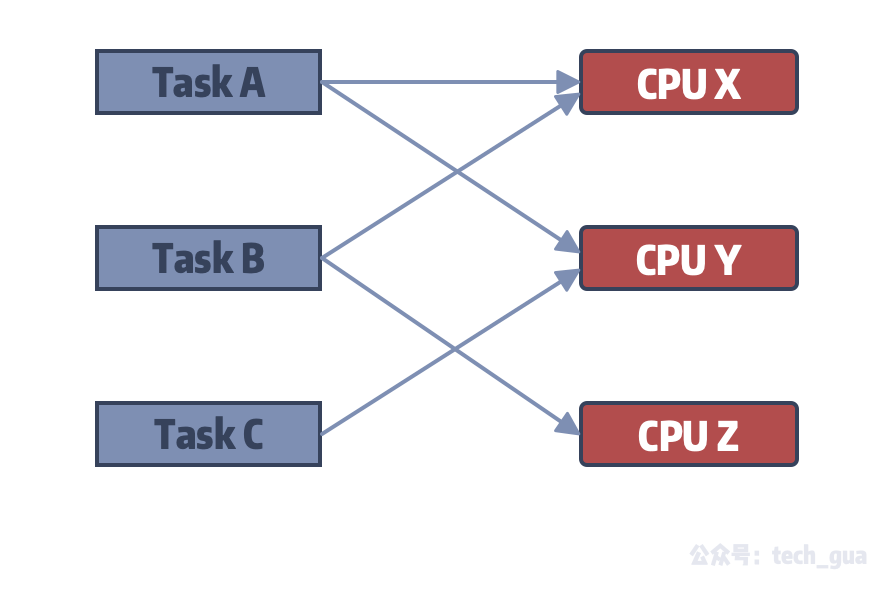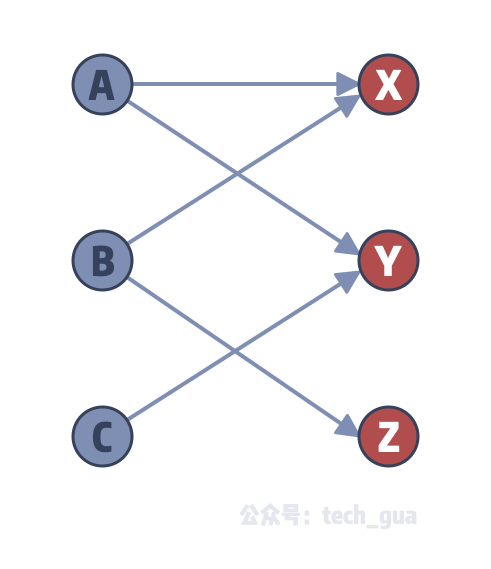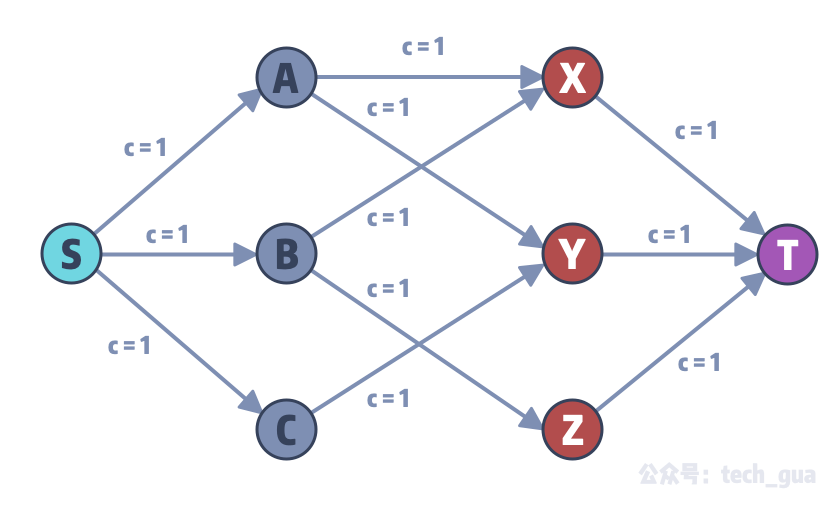# 计算机 CPU 指派问题# 问题抽象与二分图最大匹配# 思考和转化问题

## 使用结果反向启发## 赋权值量化图# FF 最大流解决二分图最大匹配问题

``````#include <iostream>
#include <string>
#include <vector>
using namespace std;

const int MAX_V = 1e4 + 5;

struct Edge {
// 终点、容量、反向边
int to, cap, rev;
Edge(int _to, int _cap, int _rev): to(_to), cap(_cap), rev(_rev) {}
};

vector<Edge> G[MAX_V];
bool used[MAX_V];

void add_edge(int from, int to, int cap) {
G[from].push_back(Edge(to, cap, G[to].size()));
G[to].push_back(Edge(from, 0, G[from].size() - 1));
}

/**
*
* @param c 当前节点
* @param t 汇点
* @param f 当前路径中的容量最小值
* @return
*/
int dfs(int c, int t, int f) {
if (c == t) {
return f;
}
used[c] = true;
for (int i = 0; i < G[c].size(); ++ i) {
Edge &e = G[c][i];
if (!used[e.to] && e.cap > 0) {
int d = dfs(e.to, t, min(f, e.cap));
if (d > 0) {
e.cap -= d;
G[e.to][e.rev].cap += d;
return d;
}
}
}
return 0;
}

int main() {
// A, B, C 为节点 0, 1, 2
// X, Y, Z 为节点 3, 4, 5
// S, T 为节点 6, 7

// 题目描述的 A, B, C 和 X, Y, Z 的关系

// 模拟超级源 S 点

// 模拟超级汇 T 点

// 求最大流
int flow = 0;
for (;;) {
memset(used, 0, sizeof(used));
int f = dfs(6, 7, 1);
if (f == 0) {
cout << flow << endl; // 3 验证通过
return 0;
}
flow += f;
}
}
``````

# 总结

“万物皆可网络流”，真正有趣的才刚刚开始！